# SSAT Elementary Level Math : How to find the part from the whole

## Example Questions

← Previous 1 3 4 5 6 7 8 9 58 59

### Example Question #1 : How To Find The Part From The Whole

Find the digit in the hundredths place.

123.4567Explanation:

The "hundredths" place is two digits to the RIGHT of the decimal.

### Example Question #1 : How To Find The Part From The Whole

Which fraction is less than?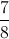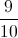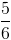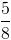Explanation:

If you think about a pie, which fraction is less or smaller thanof the pie?of a pie is less thanof a pie.  All of the other fractions are more than or greater thanof a pie.

### Example Question #1 : How To Find The Part From The Whole

What is 60% of 700?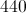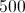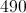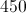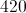Explanation:

60% of 700 can be calculated by multiplying 0.60 by 700: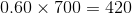### Example Question #4 : How To Find The Part From The Whole

Find the part from the whole.  What number goes in the box?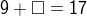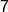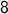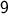Explanation:

Think: what two parts equal the whole (17)?

9 plus what equals 17?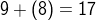### Example Question #2 : How To Find The Part From The Whole

Find the missing number.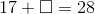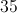Explanation:

To find the missing part, think: 17 (part) plus what (missing part) equals 28 (whole)?

We can find the missing part by using the idea that whole minus part equals the missing part.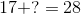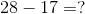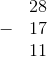So, the missing part is 11. We can check by adding the parts and getting the whole (28).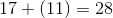### Example Question #6 : How To Find The Part From The Whole

Find the digit in the hundreds place.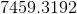Explanation:

Do not confuse hundredths place and hundreds place. The hundreds place is between the tens place and the thousands place, in front of the decimal.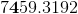### Example Question #7 : How To Find The Part From The Whole

Find the part from the whole.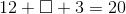Explanation:

To find a whole, add the three parts.  First, add the two parts together: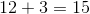Since one of the parts is still missing, think: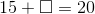15 (part) plus what (missing part) equals 20 (whole)?

The missing part is 5.

### Example Question #8 : How To Find The Part From The Whole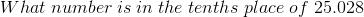Explanation: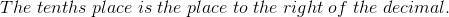### Example Question #9 : How To Find The Part From The Whole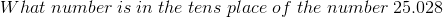Explanation: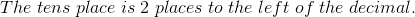### Example Question #10 : How To Find The Part From The Whole

23481.3084 Which number is in the thousands place?